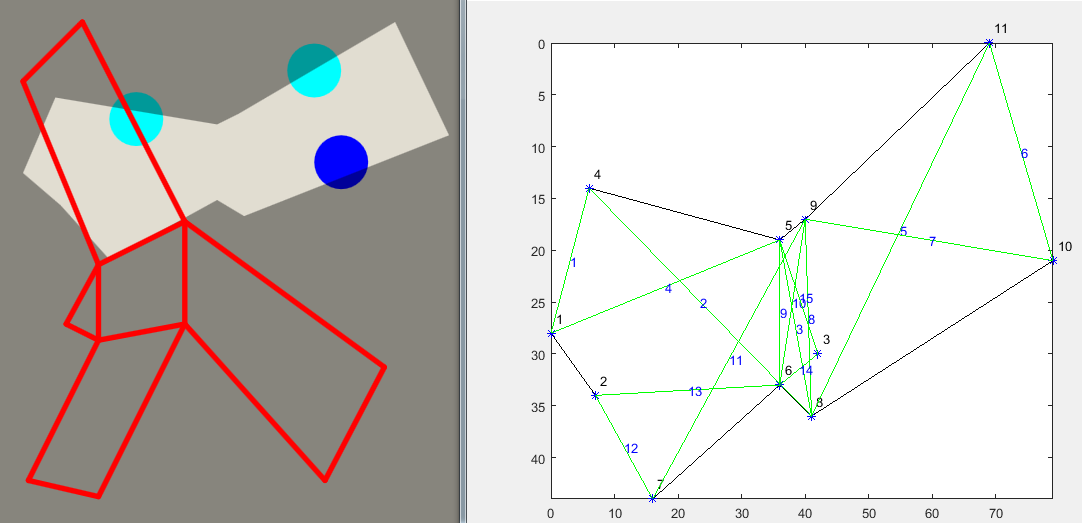# Problem 52355. ICFP2021 Hole-In-Wall: Solve Problem 47, Score=0, Figure Vertices 11, Hole Vertices 10

The ICFP held its annual 3-day contest in July 2021 with Hole-In-Wall. Contest Specification.
The contest folds the figure in Red to fit within the hole shown in light grey. A final solution is shown to aid in programming.This Challenge is to solve ICFP problems 47 according to the Specification when given the hole vertices in hxy, original figure vertices in pxy, segment matrix mseg, and epsilon. The hxy matrix is [N+1,2] where N is number of hole vertices. A repeat of the first vertex occurs for drawing the hole. The pxy(original) and npxy(final) matrices are [P,2] where P is the number of figure vertices. The mseg indicates connected vertices that must maintain a length as a function of epsilon from the original length. The final figure vertices must be integer thus the allowed fuzziness of segment lengths. Brute force of problem 47 may take 180 seconds due to the 10 hole vertices.
Valid is 1) all npxy vertices on or inside the hole, hxy 2) all lengths squared of npxy segments must match the pxy segments within an allowed epsilon, abs(Lsqr(npxy,seg(i,:))/Lsqr(pxy,seg(i,:))-1)<= epsilon/1000000. Lsqr is length squared.
Score is sum of minimum square distances to the figure from each unique hole vertex.
npxy=Solve_ICFP047(hxy, pxy, mseg, epsilon)
This challenge requires a Score of zero. The npxy vertices set must contain an nchoosek(1:nP,nP-1) permutation of the hole vertices as number of figure vertices,nP, equals hole vertices, nH, plus one. One method would be to reduce the nchoosek to force the longest figure segment to fit across a pair of hole vertices. This problem with its solution shown shows that a recursive point to available hole vertices could be a more general solution.
The function template includes routines to read ICFP problem files and to write ICFP solution files.
The ICFP 2021 Hole In Wall contest site has enabled a public user login to allow submissions. A login must be created to access all the problems and to submit solutions. Solutions are simple text files. Other challenges will show reading files, drawing figures, and producing submission files. To fully access the ICFP/Problems site use Register Team. Anyone can select Problems Page and then click problem numbers to see the puzzles and to download problem files.

### Solution Stats

100.0% Correct | 0.0% Incorrect
Last Solution submitted on Jul 22, 2021

### Community Treasure Hunt

Find the treasures in MATLAB Central and discover how the community can help you!

Start Hunting!